SEARCH HOMEMath Central Quandaries & QueriesHi! I was just wondering, how do you write the squared root of 7x(to the 5th) in exponential form? If you could help explain that I'd be grateful. Thanks CassiaHello. I believe you question looks something like this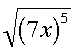When we are given questions with square roots we have to remember a little rhyme. “The number the denominator goes in the notch” Basically what that means is the number that is in the notch of the root sign becomes a fraction exponent, in our case 1/2. That may be confusing so let’s look at the example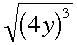If we want to put something like this into exponential form we first want to get rid of the root sign and make it into a fractional exponent. So doing that we turn the root into the fraction ½ and make that into an exponent.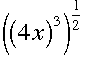then from here we want to simplify the exponents. Since we have one exponent raised to another we just need to multiply them getting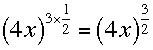. This would then be the exponential form of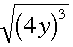We can even take it one step further and evaluate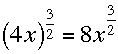Hope this helps,

Brennan YaremkoMath Central is supported by the University of Regina and The Pacific Institute for the Mathematical Sciences.